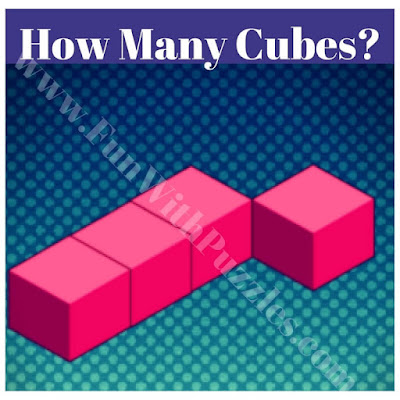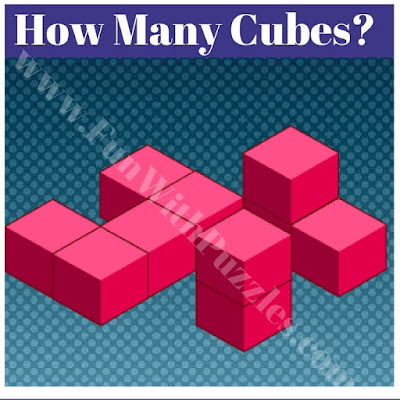I have seen many aptitude tests in which an isometric view of cubes is given in which some of the cubes are stacked over each other. One's challenge is to count the number of cubes in the given picture. These are kind of spatial puzzles that will test one's observational skills. Here are similar brain teasers in which one has to count the number of cubes in an isometric view.
In these brain teasers, some cubes are given in isometric view. One has to find how many cubes are there in the given figure. Try to count these cubes as soon as possible.
Answers to these brain teasers are given at the end. Do post your answers in the comment section.1. An easy brain teaser to count the number of cubes2. Count the number of cubes in the given figure3. Brainteaser to count the number of cubes4. How many cubes are there in the figure?5. How many cubes are there in the picture puzzle?